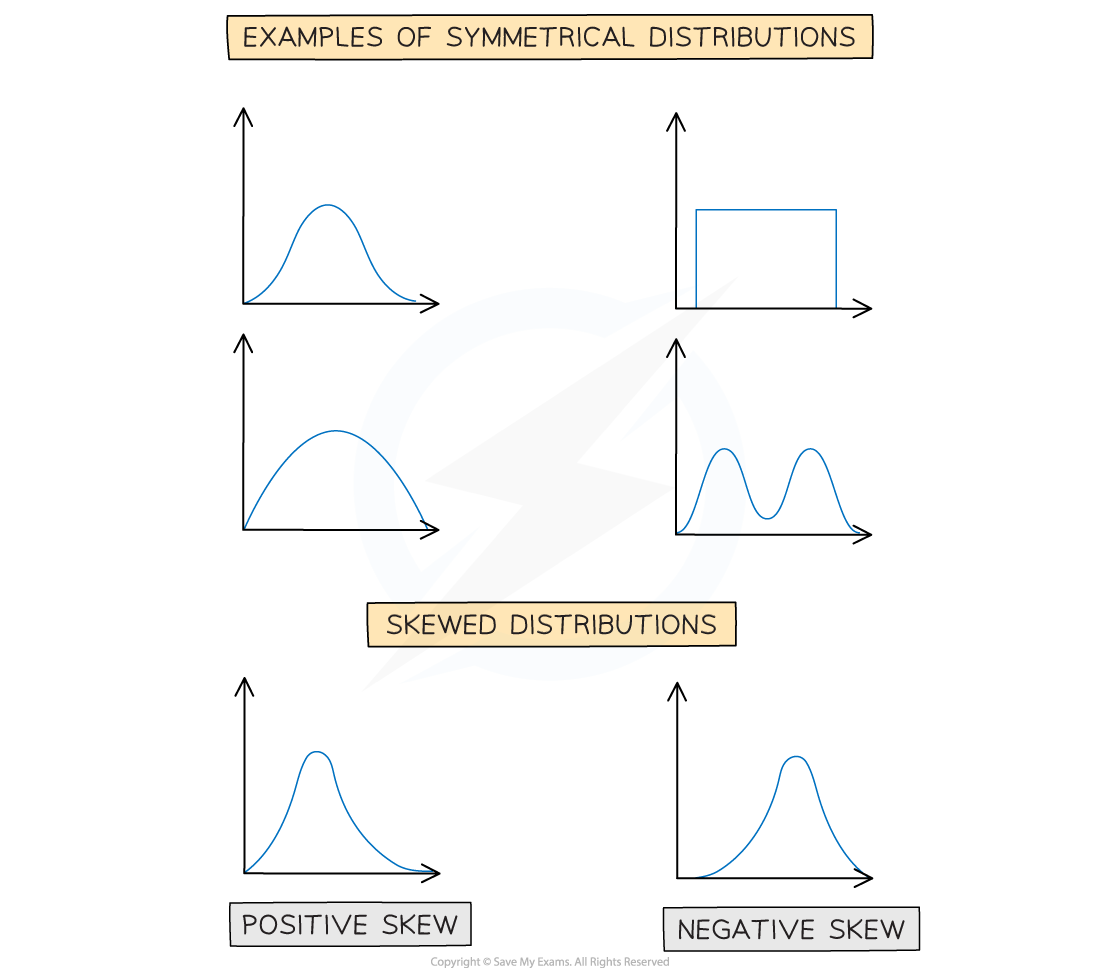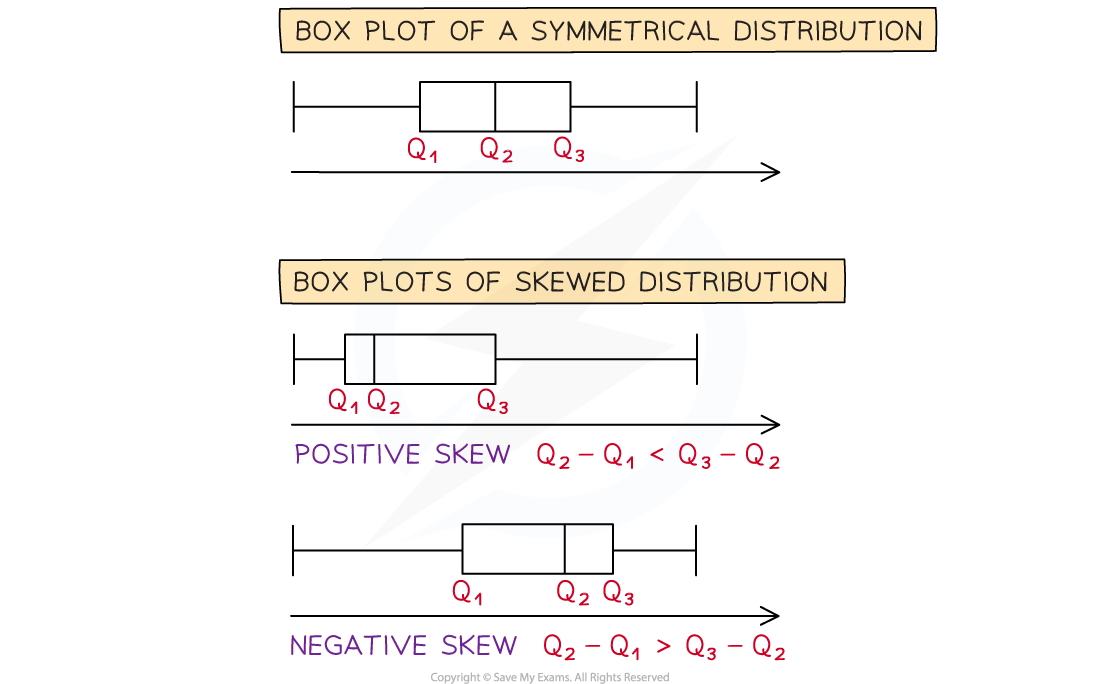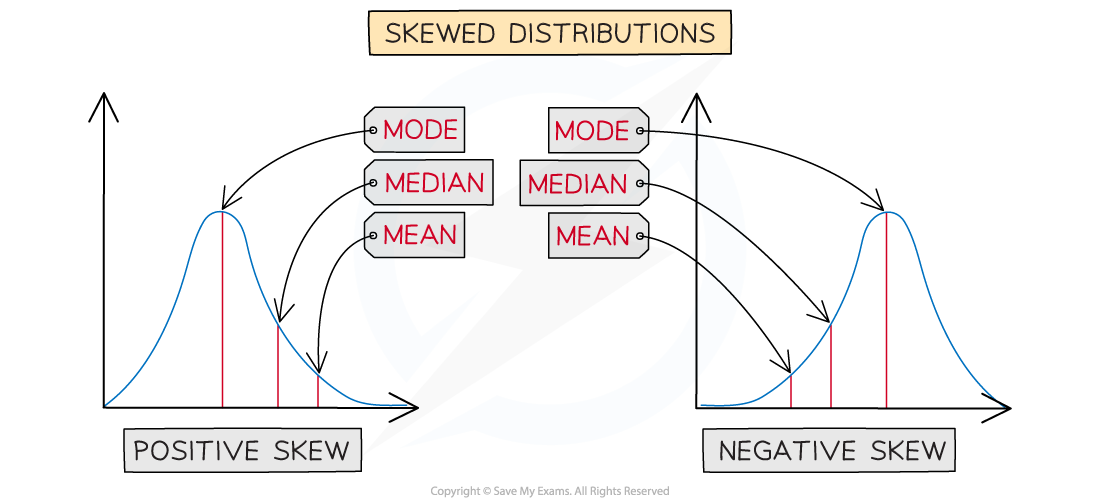# AQA A Level Maths: Statistics复习笔记2.3.3 Skewness

### Skewness

The distribution of a data set is either symmetrical or it has skewness.

#### What is skewness?

• Skewness describes the way in which data in a non – symmetrical distribution is leaning
• A distribution that has its tail on the right side has positive skew
• A distribution that has its tail on the left side has negative skew• If the distribution is shown on a box plot looking at the difference between the quartiles can help decide how it is skewed
• If the median is closer to the lower quartile then the distribution has positive skew
• Q3 -  Q2 > Q2- Q1
• If the median is closer to the upper quartile then the distribution has negative skew
• Q3 -  Q2 < Q2 - Q1• Looking at the values of the statistics can help you decide whether distribution is positively skewed or negatively skewed
• In a positively skewed distribution
• Mode < median < mean
• In a negatively skewed distribution
• Mean < median < mode#### Exam Tip

• You only need to be able to recognise the different types of skewness. It can also help to comment on skewness when asked to compare distributions.